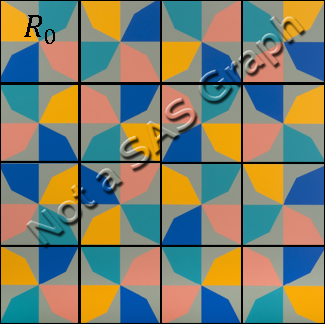In a previous article, I discussed a beautiful painting called "Phantom’s Shadow, 2018" by the Nigerian-born artist, Odili Donald Odita. I noted that if you overlay a 4 x 4 grid on the painting, then each cell contains a four-bladed pinwheel shape. The cells display rotations and reflections of the pinwheel.

The figure at the right overlays the grid on Odita's painting. The cell in the upper right (marked R0) displays one pinwheel. When I first saw Odita's artwork, I thought that all 16 cells were a rotation or reflection of this one pinwheel. However, it turns out that Odita's work contains a mathematical twist. To generate all the pinwheels in the image, you need to use two different colorings of the pinwheel. The first coloring is (in counter-clockwise order) teal, orange, blue, and salmon. The second coloring is teal, salmon, blue, and orange. You cannot get the second colored pinwheel by rotating and reflecting the first.

### Rotations and reflections of a pinwheel

The "fundamental pinwheel" (R0) is displayed to the left. As I showed in the previous article, you can generate this pinwheel by starting with a four-sided polygon and rotating it around the origin.

For simplicity, you can start with the coordinates of the pinwheel. The following SAS data set contains the coordinates of the pinwheel vertices. As shown in the previous article, you can use the POLYGON statement in PROC SGPLOT to visualize the pinwheel.

```data Shape; input ID x y @@; datalines; 0 0 0 0 0.667 0.333 0 1 1 0 0 1 0 0 0 1 0 0 1 -0.333 0.667 1 -1 1 1 -1 0 1 0 0 2 0 0 2 -0.667 -0.333 2 -1 -1 2 0 -1 2 0 0 3 0 0 3 0.333 -0.667 3 1 -1 3 1 0 3 0 0 ;```

If you ignore the colors, the pinwheel shape has rotational and reflectional symmetries. Let's understand how this pinwheel looks when it is rotated or reflected under the symmetries of the square. The symmetries make up a finite group of order eight, which is known as the dihedral group of the square or D4. The group has a representation in terms of eight 2 x 2 orthogonal matrices. The following SAS/IML program defines a function that will transform a planar set of points according to one of the matrices in the dihedral group. The program reads the pinwheel coordinates into a data matrix and transforms the pinwheel according to each element of D4. The results are plotted by using PROC SGPANEL:

```proc iml; /* actions of the D4 dihedral group */ start D4Action(v, act); /* the subroup of rotations by 90 degrees */ if act=0 then M = { 1 0, 0 1}; else if act=1 then M = { 0 -1, 1 0}; else if act=2 then M = {-1 0, 0 -1}; else if act=3 then M = { 0 1, -1 0}; /* the subgroup of reflections across horz, vert, or diagonals */ else if act=4 then M = {-1 0, 0 1}; else if act=6 then M = { 0 -1, -1 0}; else if act=7 then M = { 1 0, 0 -1}; else if act=5 then M = { 0 1, 1 0}; return( v*M` ); /* = (M*z`)` */ finish; /* read in the pinwheel shape */ use Shape; read all var {x y} into P; read all var "ID"; close; /* write out the transformation of the pinwheel under the D4 actions */ OpNames = {"Identity" "R1" "R2" "R3" "S0" "S1" "S2" "S3"}; Name = OpNames; Q = {. . .}; create Panel from Name Q[c={'Name' 'ID' 'x' 'y'}]; do i = 0 to 7; R = D4Action(P, i); Q = ID || R; Name = j(nrow(Q), 1, OpNames[i+1]); append from Name Q; end; close; QUIT;   ods graphics / width=720px height=480px; /* for convenience, define macros for the COLAXIS and ROWAXIS options */ %macro colOpts; colaxis offsetmin=0 offsetmax=0 display=(nolabel noticks novalues); %mend; %macro rowOpts; rowaxis offsetmin=0 offsetmax=0 display=(nolabel noticks novalues); %mend;   title "Dihedral Group (D4) Actions on Pinwheel Figure"; title2 "Colors=(Teal, Orange, Blue, Salmon)"; proc sgpanel data=Panel noautolegend; styleattrs wallcolor=&gray datacolors=(&teal &orange &blue &salmon); panelby Name / columns=4 onepanel; polygon x=x y=y ID=ID / group=ID fill; %colOpts; %rowOpts; run;```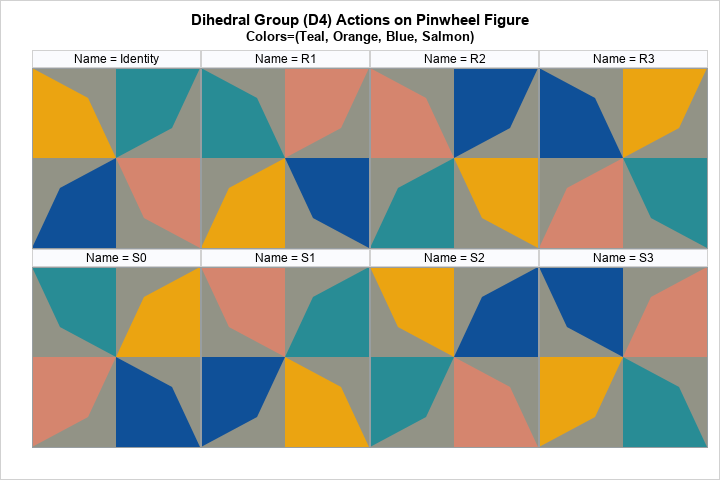The labels for each cell indicate the element of D4 that transforms the original pinwheel. The first row represents rotations by 0, 90, 180, and 270 degrees. The second row represents a vertical reflection (S0), a reflection across a diagonal line (S1), a reflection across a different diagonal (S2), and a horizontal reflection (S3). As mentioned earlier, I assumed that these eight images would be sufficient to reproduce the cells in Odita's artwork, but I was wrong. Let's look at the 16 cells in Odita's image and label each cell according to the elements of the D4 dihedral group: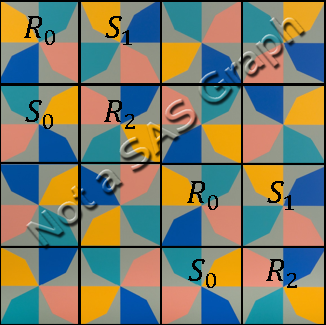I was surprised to discover that only half of the cells are transformations of the upper-left pinwheel. Furthermore, the four cells in the upper-left corner are arranged in the same order as the four cells in the lower-right corner. Out of the eight possible transformations of the pinwheel under the D4 group, Odita only uses four. The remaining cells either have the colors in a different order or have the "vane" of the pinwheel pointing in a different direction.

### A second generator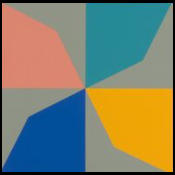Mathematically, Odita's pattern has a second pinwheel "generator." You could choose any of the unlabeled cells to be the generator of the remaining cells, but I will choose the cell on the second row and third column, which is displayed to the left.

For this pinwheel, the vanes are pointing in the same direction as the first pinwheel, but the colors (in counter-clockwise order) are teal, salmon, blue, and orange. Notice that the salmon and orange colors have switched their order.

In terms of the SAS data set, we can create this new colored pinwheel by switching the ID variable for the observations that have ID=1 and ID=3. You can then create a panel of images for this new pinwheel under the dihedral transformations, as follows:

```/* switch the colors of the 2nd and 4th vanes */ data Panel2; set Panel; if ID=1 then ID=3; else if ID=3 then ID=1; run;   title "Dihedral Group (D4) Actions on Pinwheel Figure"; title2 "Colors=(Teal, Salmon, Blue, Orange)"; proc sgpanel data=Panel2 noautolegend; styleattrs wallcolor=&gray datacolors=(&teal &orange &blue &salmon); panelby Name / columns=4 onepanel; polygon x=x y=y ID=ID / group=ID fill; %colOpts; %rowOpts; run;```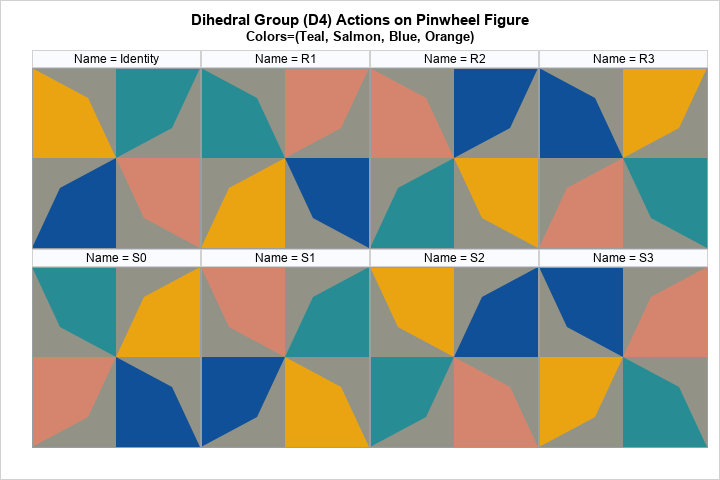Let's use a prime to distinguish the elements of this second panel. That is, the first row represents the elements R`0, R`1, R`2, and R`3. The second row represents the elements S`0, S`1, S`2, and S`3. We can use these labels to identify the remaining cells in Odita's work, as follows: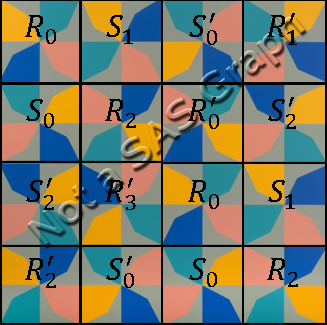The remaining eight cells display six of the possible patterns for the dihedral actions on the second generator. The S`0 and S`2 actions are repeated. The S`1 and S`3 actions do not appear.

### Using SAS to generate mathematical art

Now that we have identified all the cells in Odita's painting, we could actually use PROC SGPANEL in SAS to create a mathematical reproduction of his work. Or, we can use SAS to create new Odita-inspired images.

I will do the latter. The following statements stack the panels of the dihedral group for the two fundamental pinwheels:

```data Pinwheels; length Name \$20; set Panel Panel2; Group = floor((_N_-1)/20); run;   ods graphics / width=640px height=640px; title "Dihedral Shadow"; proc sgpanel data=Pinwheels noautolegend; styleattrs wallcolor=&gray datacolors=(&teal &orange &blue &salmon); panelby Group / columns=4 onepanel noheader; polygon x=x y=y ID=ID / group=ID fill; %colOpts; %rowOpts; run;```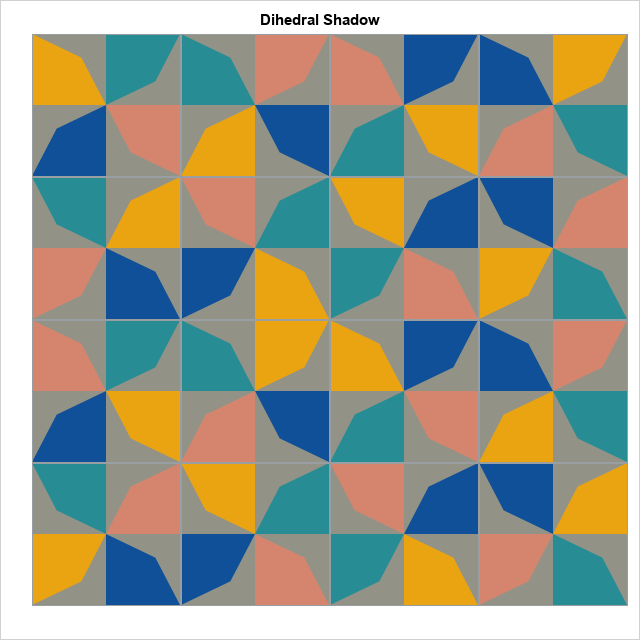In homage to Odita, I name this mathematical image "Dihedral's Shadow." The first two rows show the actions (rotations and reflections) of the D4 dihedral group on a pinwheel shape whose colors are in the sequential order "teal, orange, blue, and salmon." The last two rows show the dihedral actions on a pinwheel shape whose colors are in the order "teal, salmon, blue, and orange."

Notice that this mathematical image does not share some of the aesthetic properties of Odita's work. In Odita's image, the colors of the polygons are chosen so that the four neighbors (up-down and left-right) of a polygon are different colors from the polygon itself. My creation does not have that property. For example, if you think of the image as an 8 x 8 grid and traverse the first row, you see two teal polygons followed by two salmon polygons followed by two blue polygons. In Odita's work, no row has two adjacent polygons that have the same color.

I don't like the three orange polygons in the middle of my image. Odita arranged his pinwheel images to avoid neighboring polygons that have the same color. I suppose I could try arranging the pinwheels in a different order.

### Summary

There is much more that could be said about the mathematical structures in Odita's work, "Phantom’s Shadow, 2018." In this article, I show how you can analyze components of the painting as reflections and rotations of two four-color pinwheel-shaped figures. Mathematically, these images can be generated by using orthogonal matrices. The images can be assembled into a lattice by using PROC SGPANEL in SAS.

This article shows how to create a purely mathematical image: the dihedral actions on two pinwheel shapes. It arranges the images without consideration of how a pinwheel looks adjacent to its neighbors. In a triumph of art over mathematics, I think Odita's art is more pleasing than my imitation.

Would you like to use SAS to create mathematical art? You can download the SAS program that I used to create the images in this article. I challenge my readers to use the Pinwheels data set to create their own mathematical works. You can use one of the following ideas, or explore your own ideas:

• The design of experiments uses arrays to describe factors that are varied in the experiment. Use these pinwheel images to create a visual representation of an experimental design. The factors are rotation (4 levels), reflection (4 levels), and color-sequence (2 levels).
• Rearrange the cells in "Dihedral's Shadow" to reduce the number of adjacent polygons that have the same color. You can do this manually, but extra points if you use math or SAS/OR software to minimize the number of adjacent polygons that have the same color!
• Use a random number generation to create a random arrangement of the pinwheels.

I have created a thread on the SAS Support Communities that you can use to post your own creations. Have fun!

The post Use SAS to create mathematical art appeared first on The DO Loop.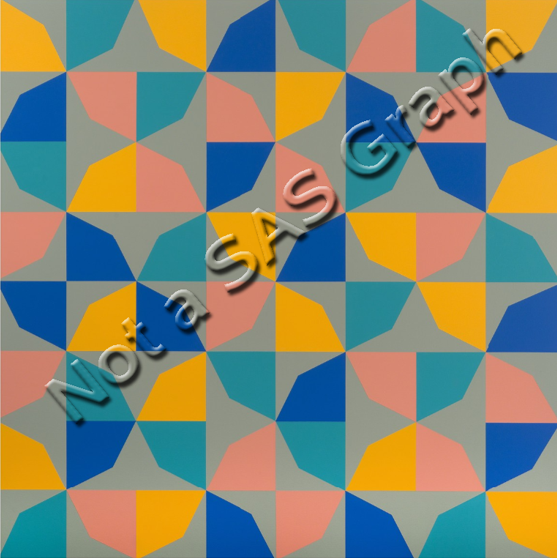Art evokes an emotional response in the viewer, but sometimes art also evokes a cerebral response. When I see patterns and symmetries in art, I think about a related mathematical object or process. Recently, a Twitter user tweeted about a painting called "Phantom’s Shadow, 2018" by the Nigerian-born artist, Odili Donald Odita. A modified version of the artwork is shown to the right.

The artwork is beautiful, but it also contains a lot of math. The image shows 64 rotations, reflections, and translations of a polygon in four colors. As I will soon explain, the image can also be viewed as 4 x 4 grid where each cell contains a four-bladed pinwheel. The grid displays rotations and reflections of a pinwheel shape.

When I saw this artwork, it inspired me to look closely at its mathematical structure and to create my own mathematical version of the artwork in SAS. This article shows how to use rotations to create a pinwheel from a polygon. A second article discusses how to use rotations and reflections to create a mathematical interpretation of Odita's painting.

### Create a polygon

Look closely at the upper left corner of "Phantom's Shadow." You will see the following pinwheel-shaped figure: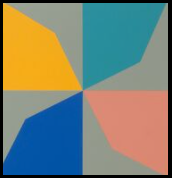If the center of the pinwheel is the origin, then this pinwheel is based on a sequence of 90-degree rotations of the teal-colored polygon about the origin. Each rotation is a different color (teal, orange, blue, and salmon) on a gray background.

You can assign coordinates to the vertices of the teal polygon. If three vertices are on the corners of the unit square, the fourth vertex appears to be at (2/3, 1/3). You can put the vertices into a SAS data set and graph the polygon by using the POLYGON statement in PROC SGPLOT:

```%let alpha = 0.333; /* Odita's work uses a vertex as (1-alpha, alpha) */ data Poly1; ID = 1; input x y @@; if x=. then do; /* just for fun, support other locations of vertex */ x = 1 - &alpha; y = &alpha; end; datalines; 0 0 . . 1 1 0 1 0 0 ;   %let blue = CX0f5098; %let orange = CXeba411; %let teal = CX288c95; %let salmon = CXd5856e; %let gray = CX929386;   ods graphics / width=480px height=480px; title "Base Polygon"; proc sgplot data=Poly1 aspect=1; styleattrs wallcolor=&gray; polygon x=x y=y ID=ID / fill fillattrs=(color=&teal) outline; xaxis offsetmin=0.005 offsetmax=0; yaxis offsetmin=0 offsetmax=0.005; run;```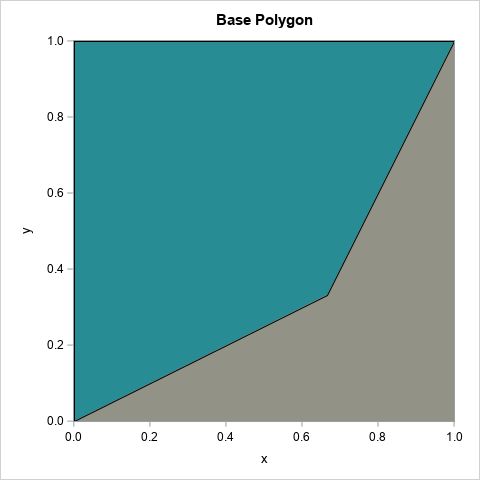The complete artwork repeats this polygon 64 times in a grid by using different colors and different orientations. But the colors and orientations are not random—although that would also look cool! Instead, there is an additional structure. The polygon is part of a pinwheel, and the pinwheel shape is rotated and reflected 16 times in a 4 x 4 grid. The next section shows how to create the pinwheel.

### Create a pinwheel

It is possible to perform 2-D rotations and reflections in the DATA step, but the rotations and reflections of a planar figure are most simply expressed in terms of 2 x 2 orthogonal matrices.

To form the pinwheel from the basic polygon, you can use a group of four matrices. All rotations are in the counter-clockwise direction about the origin:

• R0 is the identity matrix
• R1 is the matrix that rotates a point 90 degrees (about the origin)
• R2 is the matrix that rotates a point 180 degrees
• R3 is the matrix that rotates a point 270 degrees

You do not need advanced mathematics to understand rotations by 90 degrees. However, these four rotation matrices are an example of an algebraic structure called a finite group. These matrices form the cyclic group of order 4 (C4), where the group operation is matrix multiplication.

Regardless of their name, you can use these matrices to transform the polygon into a pinwheel. The following SAS/IML program reads in the base polygon and transforms it according to each of the four matrices. You can then plot the four images, each in a different color.

```/* rotate polygon about the origin to form a pinwheel */ proc iml; /* actions of the C4 cyclic group: rotations by 90 degrees */ start C4Action(v, act); if act=0 then M = { 1 0, 0 1}; else if act=1 then M = { 0 -1, 1 0}; else if act=2 then M = {-1 0, 0 -1}; else if act=3 then M = { 0 1, -1 0}; return( v*M` ); /* = (M*z`)` */ finish;   use Poly1; read all var {x y} into P; close; /* read the polygon */   /* write the pinwheel */ Q = {. . .}; create Shape from Q[c={'ID' 'x' 'y'}]; do i = 0 to 3; R = C4Action(P, i); /* rotated image of polygon */ Q = j(nrow(R), 1, i) || R; /* save the image to a data set */ append from Q; end; close; QUIT;   title "Pinwheel Figure"; title2 "Colors=(Teal, Orange, Blue, Salmon)"; proc sgplot data=Shape aspect=1; styleattrs wallcolor=&gray datacolors=(&teal &orange &blue &salmon); polygon x=x y=y ID=ID / group=ID fill; xaxis offsetmin=0 offsetmax=0; yaxis offsetmin=0 offsetmax=0; run;```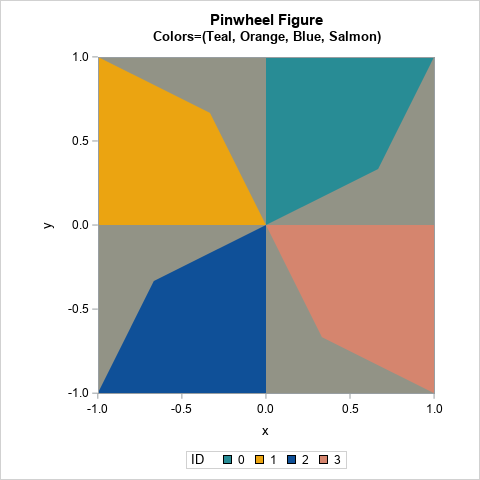The resulting image is the four-blade pinwheel, which is the basis of Odita's artwork.

### A grid of pinwheels

The rest of "Phantom’s Shadow, 2018" can be described in terms of rotations and reflections of the pinwheel shape...with a twist! The next article introduces the dihedral group of order 4 (D4). This is a finite group of order eight and is the symmetry group of the square. It turns out that you can use the elements of D4 to generate the 16 pinwheels in Odita's artwork. You can then use PROC SGPANEL in SAS to generate a graph that looks like "Phantom's Shadow," or you can create your own Odita-inspired mathematical artwork! Stay tuned!

The post The art of rotations and reflections appeared first on The DO Loop.Most games of skill are transitive. If Player A wins against Player B and Player B wins against Player C, then you expect Player A to win against Player C, should they play. Because of this, you can rank the players: A > B > C

Interestingly, not all games are transitive. The game "Rock, Paper, Scissors," is an intransitive game. In this game, Rock beats Scissors, Scissors beats Paper, and Paper beats Rock. You cannot rank the game shapes. You cannot declare that one shape wins more often than another.

In "Rock, Paper, Scissors," both players display their shapes simultaneously. If not, the second player could win 100% of the time by knowing the shape that is shown by the first player.

### Intransitive dice

Recently, I saw an intransitive game that involves special six-sided dice. In this game, there are four dice: A, B, C, and D. Two players each choose a die and roll it. The higher number wins. If the players choose their die sequentially, the second player is at a huge advantage: No matter which die the first player chooses, the second player can choose a die such that his probability of winning the game is 2/3.

Clearly, the numbers on the faces of the dice are important since this advantage does not exist for the usual six-sided dice. One set of intransitive dice have faces as follows:
A = {0, 0, 4, 4, 4, 4}
B = {3, 3, 3, 3, 3, 3}
C = {2, 2, 2, 2, 6, 6}
D = {1, 1, 1, 5, 5, 5}

For these dice:

• A wins against B with probability 2/3.
• B wins against C with probability 2/3.
• C wins against D with probability 2/3.
• D wins against A with probability 2/3.

The probability of winning for pairs that involve die B are easy to compute because that die has a 3 on every face. By inspection, die A will win against B whenever A shows a 4, which occurs 2/3 of the time. Similarly, die B wins against die C whenever C shows a 2, which is 2/3 of the time.

The remaining probabilities can be computed by using a tree diagram for conditional probability. A tree diagram for the dice C and D are shown below. If C shows a 6 (which happens 1/3 of the time), then C wins. If C shows a 2 (which happens 2/3 of the time), then the game depends on the roll of D. If D rolls a 1 (which happens 1/2 of the time), then C wins. But if D rolls a 5 (which happens 1/2 of the time), then C loses. Since the rolls are independent, you multiply the individual probabilities to obtain the total probability of each pair of events. For C versus D, C wins with probability 2/3.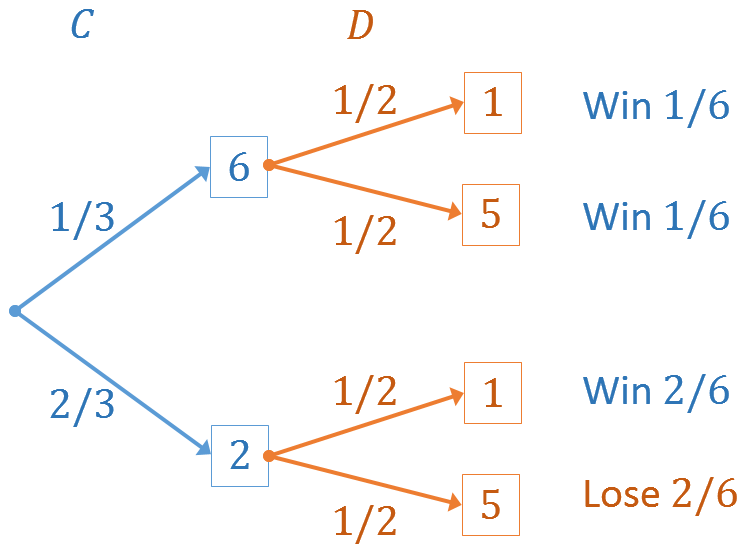You can construct a similar diagram for the D versus A game. It is also interesting to compute the probability of A versus C and B versus D for these dice.

### Simulate many games

Because conditional probability can be tricky, I always like to check my computations by running a simulation. The following SAS/IML program uses the SAMPLE function to simulate 10,000 rolls of each die. The estimated probability for winning is the number of times that one die showed the greater number, divided by 10,000, which is the total number of simulated games. For each of the four games considered, the estimated probability is approximately 2/3.

```proc iml; /* define the dice */ A = {0, 0, 4, 4, 4, 4}; B = {3, 3, 3, 3, 3, 3}; C = {2, 2, 2, 2, 6, 6}; D = {1, 1, 1, 5, 5, 5};   call randseed(1234); N = 10000; /* simulate N rolls for each die */ sA = sample(A, N); sB = sample(B, N); sC = sample(C, N); sD = sample(D, N);   /* estimate the probability of 'A vs B', 'B vs C', etc */ AvsB = sum(sA > sB) / N; BvsC = sum(sB > sC) / N; CvsD = sum(sC > sD) / N; DvsA = sum(sD > sA) / N; print AvsB BvsC CvsD DvsA;```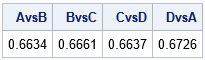There are other examples of intransitive dice. The ones in this article are called "Efron's Dice" because they were discovered by Brad Efron in 1970. Yes, the same Brad Efron who also invented bootstrap resampling methods! The following articles provide more examples and more explanation.

• "Nontransitive dice", Wikipedia article with lots of examples and a section devoted to Efron's dice, as used in this article.
• Grimes, J., (2010) "Curious Dice," Plus Magazine. A wonderful exposition.

The post Intransitive dice appeared first on The DO Loop.In the paper "Tips and Techniques for Using the Random-Number Generators in SAS" (Sarle and Wicklin, 2018), I discussed an example that uses the new STREAMREWIND subroutine in Base SAS 9.4M5. As its name implies, the STREAMREWIND subroutine rewinds a random number stream, essentially resetting the stream to the beginning. I struggled to create a compelling example for the STREAMREWIND routine because using the subroutine "results in dependent streams of numbers" and because "it is usually not necessary in simulation studies" (p. 12). Regarding an application, I asserted that the subroutine "is convenient for testing."

But recently I was thinking about two-factor authentication and realized that I could use the STREAMREWIND subroutine to emulate generating a random token that changes every 30 seconds. I think it is a cool example, and it gives me the opportunity to revisit some of the newer features of random-number generation in SAS, including new generators and random number keys.

### A brief overview of two-factor authentication

I am not an expert on two-factor authentication (TFA), but I use it to access my work computer, my bank accounts, and other sensitive accounts. The main idea behind TFA is that before you can access a secure account, you must authenticate yourself in two ways:

• Provide information that depends on a physical device that you own and that you have previously registered.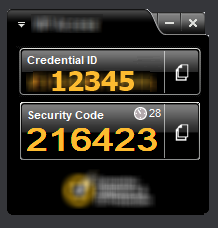Most people use a smartphone as the physical device, but it can also be a PC or laptop. If you do an internet search for "two factor authentication tokens," you can find many images like the one on the right. This is the display from a software program that runs on a PC, laptop, or phone. The "Credential ID" field is a long string that is unique to each device. (For simplicity, I've replaced the long string with "12345.") The "Security Code" field displays a pseudorandom number that changes every 30 seconds. The Security Code depends on the device and on the time of day (within a 30-second interval). In the image, you can see a small clock and the number 28, which indicates that the Security Code will be valid for another 28 seconds before a new number is generated.

After you provide a valid username and password, the account challenges you to type in the current Security Code for your registered device. When you submit the Security Code, the remote server checks whether the code is valid for your device and for the current time of day. If so, you can access your account.

### Two-factor random number streams

I love the fact that the Security Code is pseudorandom and yet verifiable. And it occurred to me that I can use the main idea of TFA to demonstrate some of the newer features in the SAS random number generators (RNGs).

Long-time SAS programmers know that each stream is determined by a random number seed. But a newer feature is that you can also set a "key" for a random number stream. For several of the new RNGs, streams that have the same seed but different keys are independent. You can use this fact to emulate the TFA app:

• The Credential ID (which is unique to each device) is the "seed" for an RNG.
• The time of day is the "key" for an RNG. Because the Security Code must be valid for 30 seconds, round the time to the nearest 30-second boundary.
• Usually each call to the RAND function advances the state of the RNG so that the next call to RAND produces a new pseudorandom number. For this application, we want to get the same number for any call within a 30-second period. One way to do this is to reset the random number stream before each call so that RAND always returns the FIRST number in the stream for the (seed, time) combination.

### Using a key to change a random-number stream

Before worrying about using the time of day as the key value, let's look at a simpler program that returns the first pseudorandom number from independent streams that have the same seed but different key values. I will use PROC FCMP to write a function that can be called from the SAS DATA step. Within the DATA step, I set the seed value and use the "Threefry 2" (TF2) RNG. I then call the Rnd6Int function for six different key values.

```proc fcmp outlib=work.TFAFunc.Access; /* this function sets the key of a random-numbers stream and returns the first 6-digit pseudorandom number in that stream */ function Rnd6Int(Key); call stream(Key); /* set the Key for the stream */ call streamrewind(Key); /* rewind stream with this Key */ x = rand("Integer", 0, 999999); /* first 6-digit random number in stream */ return( x ); endsub; quit;   options cmplib=(work.TFAFunc); /* DATA step looks here for unresolved functions */ data Test; DeviceID = 12345; /* ID for some device */ call streaminit('TF2', DeviceID); /* set RNG and seed (once per data step) */ do Key = 1 to 6; SecCode = Rnd6Int(Key); /* get random number from seed and key values */ /* Call the function again. Should produce the same value b/c of STREAMREWIND */ SecCodeDup = Rnd6Int(Key); output; end; keep DeviceID Key SecCode:; format SecCode SecCodeDup Z6.; run;   proc print data=Test noobs; run;```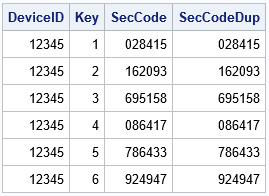Each key generates a different pseudorandom six-digit integer. Notice that the program calls the Rnd6Int function twice for each seed value. The function returns the same number each time because the random number stream for the (seed, key) combination gets reset by the STREAMREWIND call during each call. Without the STREAMREWIND call, the function would return a different value for each call.

### Using a time value as a key

With a slight modification, the program in the previous section can be made to emulate the program/app that generates a new TFA token every 30 seconds. However, so that we don't have to wait so long, the following program sets the time interval (the DT macro) to 10 seconds instead of 30. Instead of talking about a 30-second interval or a 10-second interval, I will use the term "DT-second interval," where DT can be any time interval.

The program below gets the "key" by looking at the current datetime value and rounding it to the nearest DT-second interval. This value (the RefTime variable) is sent to the Rnd6Int function to generate a pseudorandom Security Code. To demonstrate that the program generates a new Security Code every DT seconds, I call the Rnd6Int function 10 times, waiting 3 seconds between each call. The results are printed below:

```%let DT = 10; /* change the Security Code every DT seconds */   /* The following DATA step takes 30 seconds to run because it performs 10 iterations and waits 3 secs between iterations */ data TFA_Device; keep DeviceID Time SecCode; DeviceID = 12345; call streaminit('TF2', DeviceID); /* set the RNG and seed */ do i = 1 to 10; t = datetime(); /* get the current time */ /* round to the nearest DT seconds and save the "reference time" */ RefTime = round(t, &DT); SecCode = Rnd6Int(RefTime); /* get a random Security Code */ Time = timepart(t); /* output only the time */ call sleep(3, 1); /* delay 3 seconds; unit=1 sec */ output; end; format Time TIME10. SecCode Z6.; run;   proc print data=TFA_Device noobs; var DeviceId Time SecCode; run;```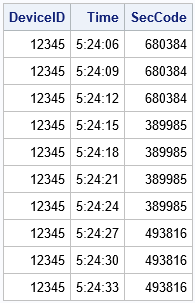The output shows that the program generated three different Security Codes. Each code is constant for a DT-second period (here, DT=10) and then changes to a new value. For example, when the seconds are in the interval [05, 15), the Security Code has the same value. The Security Code is also constant when the seconds are in the interval [15, 25) and so forth. A program like this emulates the behavior of an app that generates a new pseudorandom Security Code every DT seconds.

### Different seeds for different devices

For TFA, every device has a unique Device ID. Because the Device ID is used to set the random number seed, the pseudorandom numbers that are generated on one device will be different than the numbers generated on another device. The following program uses the Device ID as the seed value for the RNG and the time of day for the key value. I wrapped a macro around the program and called it for three hypothetical values of the Device ID.

```%macro GenerateCode(ID, DT); data GenCode; keep DeviceID Time SecCode; format DeviceID 10. Time TIME10. SecCode Z6.; DeviceID = &ID; call streaminit('TF2', DeviceID); /* set the seed from the device */ t = datetime(); /* look at the current time */ /* round to the nearest DT seconds and save the "reference time" */ RefTime = round(t, &DT); /* round to nearest DT seconds */ SecCode = Rnd6Int(RefTime); /* get a random Security Code */ Time = timepart(t); /* output only the time */ run;   proc print data=GenCode noobs; run; %mend;   /* each device has a unique ID */ %GenerateCode(12345, 30); %GenerateCode(24680, 30); %GenerateCode(97531, 30);```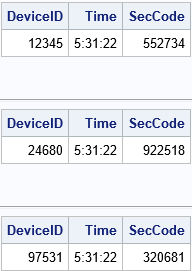As expected, the program produces different Security Codes for different Device IDs, even though the time (key) value is the same.

### Summary

In summary, you can use features of the SAS random number generators in SAS 9.4M5 to emulate the behavior of a TFA token generator. The SAS program in this article uses the Device ID as the "seed" and the time of day as a "key" to select an independent stream. (Round the time into a certain time interval.) For this application, you don't want the RAND function to advance the state of the RNG, so you can use the STREAMREWIND call to rewind the stream before each call. In this way, you can generate a pseudorandom Security Code that depends on the device and is valid for a certain length of time.

The post Rewinding random number streams: An application appeared first on The DO Loop.What is this math good for, anyway?
–Every student, everywhere

I am a professional applied mathematician, yet many of the mathematical and statistical techniques that I use every day are not from advanced university courses but are based on simple ideas taught in high school or even in grade school. I've written many blog posts in which the solution to an interesting problem requires little more than high-school math. Even when the solution requires advanced techniques, high-school math often provides the basis for solving the problem.

In celebration of the upcoming school year, here are 12 articles that show connections between advanced topics and mathematical ideas that are introduced in high-school or earlier. If you have a school-age child, read some of the articles to prepare yourself for the inevitable mid-year whine, "But, Mom/Dad, when will I ever use this stuff?!"

Obviously, most adults use basic arithmetic, fractions, decimals, and percents, but here are some less obvious uses of grade-school math:

### High-school math

Algebra, linear transformations, geometry, and trigonometry are the main topics in high school mathematics. These topics are the bread-and-butter of applied mathematics:

• Linear transformations: Anytime you create a graph on the computer screen, a linear transformation transforms the data from physical units (weight, cost, time) into pixel values. Although modern graphical software performs that linear transformation for you, a situation in which you have to manually apply a linear transformation is when you want to display data in two units (pounds and kilograms, dollars and Euros, etc). You can use a simple linear transformation to align the tick labels on the two axes.
• Intersections: In high school, you learn to compute the intersection between two lines. You can extend the problem to find the intersection of two line segments. I needed that result to solve a problem in probability theory.
• Solve a system of equations: In Algebra II, you learn to solve a system of equations. Solving linear systems is the foundation of linear algebra. Solving nonlinear systems is among the most important skills in applied mathematics.
• Find the roots of a nonlinear equation: Numerically finding the roots of a function is taught in pre-calculus. It is the basis for all "inverse problems" in which you want to find inputs to a function that produce a specified output. In statistics, a common "inverse problem" is finding the quantile of a cumulative probability distribution.
•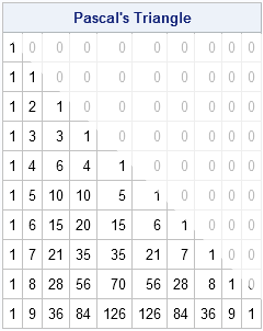• Binomial coefficients: In algebra, many teachers use the mnemonic FOIL (First, Outer, Inner, Last) to teach students how to compute the quadratic expansion of (a + b)2. Later, students learn the binomial expansion of an arbitrary power, (a + b)n. The coefficients in this expansion are called the binomial coefficients and appear in Pascal's triangle as well as in many discrete probability distributions such as the negative binomial and hypergeometric distributions.
• Pythagorean triples: In trigonometry, a huge number of homework problems involve using right triangles with side lengths that are proportional to (3, 4, 5) and (5, 12, 13). These are two examples of Pythagorean triples: right triangles whose side lengths are all integers. It turns out that you can use linear transformations to generate all primitive triples from the single triple (3, 4, 5). A high school student can understand this process, although the process is most naturally expressed in terms of matrix multiplication, which is not always taught in high school.

### High-school statistics

Many high schools offer a unit on probability and statistics, and some students take AP Statistics.

Einstein famously said, "everything should be made as simple as possible, but not simpler." It is surprising to me how often an advanced technique can be simplified and explained by using elementary math. I don't claim that "everything I needed to know about math I learned in kindergarten," but I often return to elementary techniques when I describe how to solve non-elementary problems.

What about you? What are some elementary math or statistics concepts that you use regularly in your professional life? Are there fundamental topics that you learned in high school that are deeper and more useful than you realized at the time? Leave a comment.

The post The math you learned in school: Yes, it’s useful! appeared first on The DO Loop.What is this math good for, anyway?
–Every student, everywhere

I am a professional applied mathematician, yet many of the mathematical and statistical techniques that I use every day are not from advanced university courses but are based on simple ideas taught in high school or even in grade school. I've written many blog posts in which the solution to an interesting problem requires little more than high-school math. Even when the solution requires advanced techniques, high-school math often provides the basis for solving the problem.

In celebration of the upcoming school year, here are 12 articles that show connections between advanced topics and mathematical ideas that are introduced in high-school or earlier. If you have a school-age child, read some of the articles to prepare yourself for the inevitable mid-year whine, "But, Mom/Dad, when will I ever use this stuff?!"

Obviously, most adults use basic arithmetic, fractions, decimals, and percents, but here are some less obvious uses of grade-school math:

### High-school math

Algebra, linear transformations, geometry, and trigonometry are the main topics in high school mathematics. These topics are the bread-and-butter of applied mathematics:

• Linear transformations: Anytime you create a graph on the computer screen, a linear transformation transforms the data from physical units (weight, cost, time) into pixel values. Although modern graphical software performs that linear transformation for you, a situation in which you have to manually apply a linear transformation is when you want to display data in two units (pounds and kilograms, dollars and Euros, etc). You can use a simple linear transformation to align the tick labels on the two axes.
• Intersections: In high school, you learn to compute the intersection between two lines. You can extend the problem to find the intersection of two line segments. I needed that result to solve a problem in probability theory.
• Solve a system of equations: In Algebra II, you learn to solve a system of equations. Solving linear systems is the foundation of linear algebra. Solving nonlinear systems is among the most important skills in applied mathematics.
• Find the roots of a nonlinear equation: Numerically finding the roots of a function is taught in pre-calculus. It is the basis for all "inverse problems" in which you want to find inputs to a function that produce a specified output. In statistics, a common "inverse problem" is finding the quantile of a cumulative probability distribution.
•• Binomial coefficients: In algebra, many teachers use the mnemonic FOIL (First, Outer, Inner, Last) to teach students how to compute the quadratic expansion of (a + b)2. Later, students learn the binomial expansion of an arbitrary power, (a + b)n. The coefficients in this expansion are called the binomial coefficients and appear in Pascal's triangle as well as in many discrete probability distributions such as the negative binomial and hypergeometric distributions.
• Pythagorean triples: In trigonometry, a huge number of homework problems involve using right triangles with side lengths that are proportional to (3, 4, 5) and (5, 12, 13). These are two examples of Pythagorean triples: right triangles whose side lengths are all integers. It turns out that you can use linear transformations to generate all primitive triples from the single triple (3, 4, 5). A high school student can understand this process, although the process is most naturally expressed in terms of matrix multiplication, which is not always taught in high school.

### High-school statistics

Many high schools offer a unit on probability and statistics, and some students take AP Statistics.

Einstein famously said, "everything should be made as simple as possible, but not simpler." It is surprising to me how often an advanced technique can be simplified and explained by using elementary math. I don't claim that "everything I needed to know about math I learned in kindergarten," but I often return to elementary techniques when I describe how to solve non-elementary problems.

What about you? What are some elementary math or statistics concepts that you use regularly in your professional life? Are there fundamental topics that you learned in high school that are deeper and more useful than you realized at the time? Leave a comment.

The post The math you learned in school: Yes, it’s useful! appeared first on The DO Loop.It's time to celebrate Pi Day! Every year on March 14th (written 3/14 in the US), math-loving folks celebrate "all things pi-related" because 3.14 is the three-decimal approximation to the mathematical constant, π. Although children learn that pi is approximately 3.14159..., the actual definition of π is the ratio of a circle's circumference to its diameter. Equivalently, it is distance around half of the unit circle. (The unit circle has a unit radius, so its diameter is 2.) The value for pi, therefore, depends on the definition of a circle.

But we all know what a circle looks like, don't we? How can there be more than one circle?

### Generalizing the circle

A circle is defined as the locus of points in the plane that are a given distance from a given point. This definition depends on the definition of a "distance," and it turns out that there are infinitely many ways to measure the distance between two points in the plane. The Euclidean distance between two points is the most familiar distances, but there are other definitions. For two points a = (x1, y1) and b = (x2, y2), you can define the "Lp distance" between a and b by the formula
Dp = ( |x1 – x2|p + |y1 – y2|p )1/p
This definition defines a distance metric for every value of p ≥ 1. If you set p=2 in the formula, you get the usual L2 (Euclidean) distance. If you set p=1, you get the L1 metric, which is known as the "taxicab" or "city block" distance.

You might think that the Euclidean distance is the only relevant distance, but it turns out that some of these other distances have practical applications in statistics, machine learning, linear algebra, and many fields of applied mathematics. For example, the 2-norm (L2) distance is used in least-squares regression whereas the 1-norm (L1) distance is used in robust regression and quantile regression. A combination of the two distances is used for ridge regression, LASSO regression, and "elastic net" regression.

Here's the connection to pi: If you can define infinitely many distance formulas, then there are infinitely many unit circles, one for each value of p ≥ 1. And if there are infinitely many circles, there might be infinitely many values of pi. (Spoiler alert: There are!)

### Would the real circle please stand up?

You can easily solve for y as a function of x and draw the unit circle for a representative set of values for p. The following graph was generated by the SAS step and PROC SGPLOT. You can download the SAS program that generates the graphs in this article.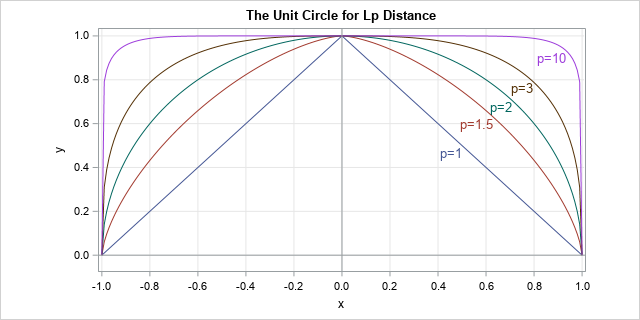The L1 unit circle is a diamond (the top half is shown), the L2 unit circle is the familiar round shape, and as p gets large the unit circle for the Lp distance approaches the boundary of the square defined by the four points (±1, ±1). For more information about Lp circles and metrics, see the Wikipedia article "Lp Space: The p-norm in finite dimensions."

Here comes the surprise: Just as each Lp metric has its own unit circle, each metric has its own numerical value for pi, which is the length of the unit semicircle as measured by that metric.

### π(p): The length of the unit semicircle for the Lp distance metric

So far, we've only used geometry, but it's time to use a little calculus. This presentation is based on Keller and Vakil (2009, p. 931-935), who give more details about the formulas in this section.

For a curve that is represented as a graph (y as a function of x), you can obtain the length of the curve by integrating the arclength. In Calculus 2, the arclength formula is derived for Euclidean distance, but it is straightforward to give the formula for the Lp distance:
s(p) = ∫ (1 + |dy/dx|p)1/p dx

To obtain a value for pi in the Lp metric, you can integrate the arclength for the upper half of the Lp unit circle. Equivalently, by symmetry, you can integrate one-eighth of the unit circle and multiply by 4. A convenient choice for the limits of integration is [0, 2-1/p] because 2-1/p is the x value where the 45-degree line intersects the unit circle for the Lp metric.

Substituting for the derivative gives the following formula (Keller and Vakil, 2009, p. 932):
π(p) = 4 ∫ (1 + u(x))1/p dx, where u(x) = |x-p - 1|1-p and the interval of integration is [0, 2-1/p].

### A pi for each Lp metric

For each value of p, you get a different value for pi. You can use your favorite numerical integration routine to approximate π(p) by integrating the formula for various values of p ≥ 1. I used SAS/IML, which supports the QUAD function for numerical integration. The arclength computation for a variety of values for p is summarized by the following graph. The graph shows the computation of π(p), which is the length of the semicircle in the Lp metric, versus values of p for p in [1, 11].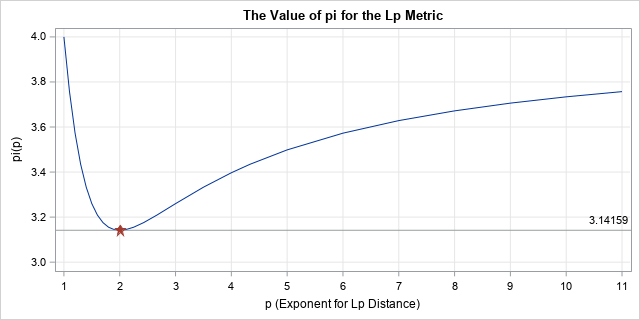The graph shows that the L1 value for pi is 4. The value decreases rapidly as p approaches 2 and reaches a minimum value when p=2 and the value of pi is 3.14159.... For p > 2, the graph of π(p) increases slowly. You can show that π(p) asymptotically approaches the value 4 as p approaches infinity.

On Pi Day, some places have contests to see who can recite the most digits of pi. I encourage you to enter the contest and say "Pi, in the L1 metric, is FOUR point zero, zero, zero, zero, ...." If they refuse to give you the prize, tell them to read this article! 😉

### Reflections on pi

One the one hand, this article shows that there is nothing special about the value 3.14159.... For an Lp metric, the ratio of the circumference of a circle to its diameter can be any value between π and 4. On the other hand, the graph shows that π is the unique minimizer of the graph. Among an infinitude of circles and metrics, the well-known Euclidean distance is the only Lp metric for which pi is 3.14159....

If you ask me, our value of π is special, without a doubt!

### References

The post The value of pi depends on how you measure distance appeared first on The DO Loop.The best way to spread Christmas cheer
is singing loud for all to hear!
-Buddy in Elf

In the Christmas movie Elf (2003), Jovie (played by Zooey Deschanel) must "spread Christmas cheer" to help Santa. She chooses to sing "Santa Claus is coming to town," and soon all of New York City is singing along.

The best sing-along songs are short and have lyrics that repeat. Jovie's choice, "Santa Claus is coming to town," satisfies both criteria. The musical structure of the song is simple:

• Verse 1: You better watch out / You better not cry / Better not pout / I'm telling you why
• Tag line: Santa Claus is coming to town
• Verse 2: He's making a list / And checking it twice; / Gonna find out / Who's naughty and nice
• Tag line repeats
• Bridge: He sees you when you're sleeping / He knows when you're awake / He knows if you've been bad or good / So be good for goodness sake! / O!
• Verse 1 repeats
• Partial tags and final tag: Santa Claus is coming / Santa Claus is coming / Santa Claus is coming to town

There is a fun way to visualize repetition in song lyrics. For a song that has N words, you can define the repetition matrix to be the N x N matrix where the (i,j)th cell has the value 1 if the i_th word is the same as the j_th word. Otherwise, the (i,j)th cell equals 0. You can visualize the matrix by using a two-color heat map. Colin Morris has a web site devoted to these visualizations.

The following image visualizes the lyrics of "Santa Claus is coming to town." I have added some vertical and horizontal lines to divide the lyrics into seven sections: the verses (V1 and V2), the tag line (S), and the bridge (B).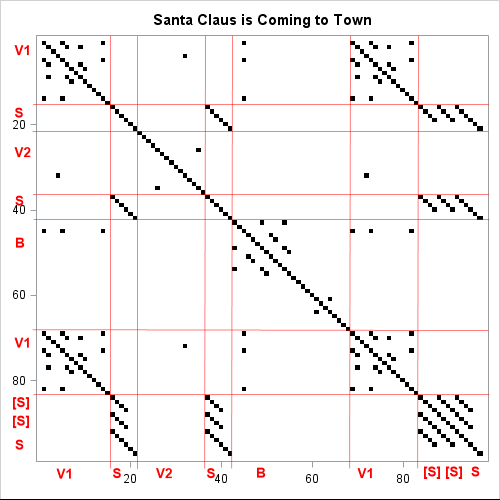The image shows the structure of the repetition in the song lyrics:

• The first verse contains the repetition of the words 'you', 'better', and 'not'.
• The second verse repeats only the word 'out' from Verse 1.
• The bridge repeats the word 'you', which appeared three times in Verse 1. It also repeats several words ('when', 'knows', 'good', ...) within the bridge.
• The tag line "Santa Claus is coming [to town]" is repeated a total of five times.

Now that you understand what a repetition matrix looks like and how to interpret it, let's visualize a few other classic Christmas songs that contain repetitive lyrics! To help "spread Christmas cheer," I'll use shades of red and green to visualize the lyrics, rather than the boring white and black colors.

### The Twelve Days of Christmas

If you make a list of Christmas songs that have repetition, chances are "The Twelve Days of Christmas" will be at the top of the list. The song is formulaic: each new verse adds a few new words before repeating the words from the previous verse. As a result, the repetition matrix is almost boring in its regularity. Here is the visualization of the classic song (click to enlarge):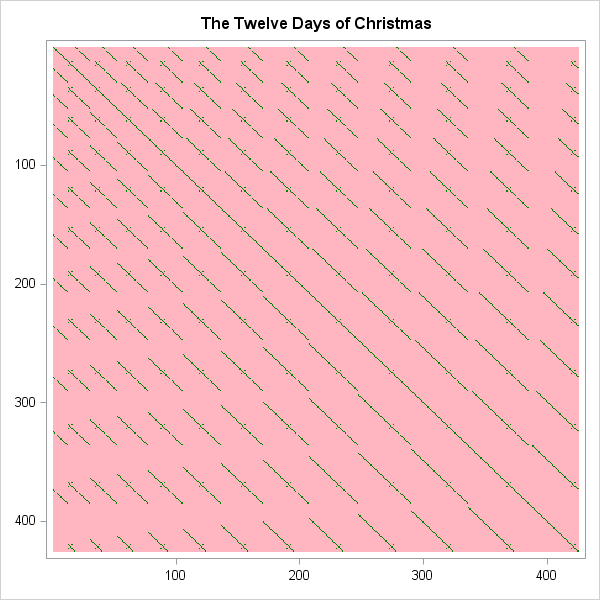### Little Drummer Boy

Another highly repetitive Christmas song is "The Little Drummer Boy," which features an onomatopoeic phrase (Pa rum pum pum pum) that alternates with the other lyrics. A visualization of the classic song is shown below: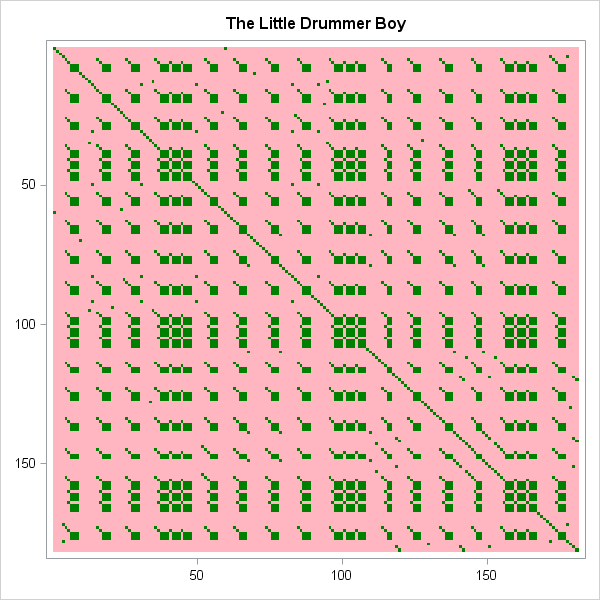### Silver Bells

In addition to repeating the title, "Silver Bells" repeats several phrases. Most notably, the phrase "Soon it will be Christmas Day" is repeated multiple times at the end of the song. Because only certain phrases are repeated, the visualization has a pleasing structure that complements the song's lyrical qualities: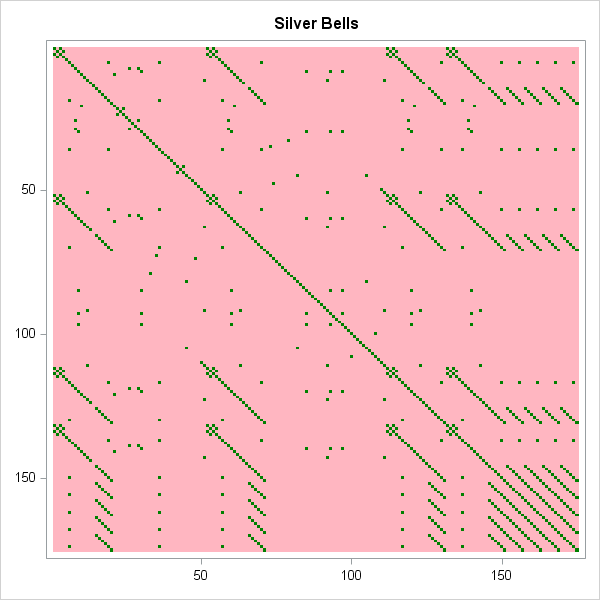### Silent Night

To contrast the hustle, bustle, and commercialism of Christmas, I enjoy hearing songs that are musically simple. One of my favorites is "Silent Night." Each verse is distinct, yet each begins with "Silent night, holy night!" and ends by repeating a phrase. The resulting visualization is devoid of clutter. It is visually empty and matches the lyrical imagery, "all is calm, all is bright."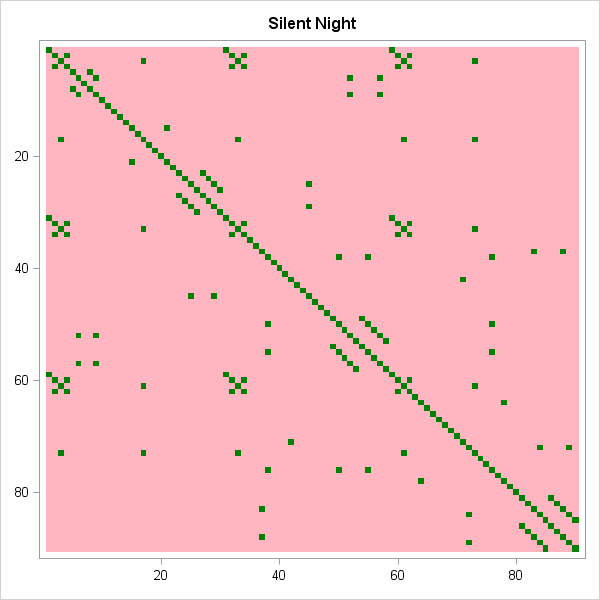You can download the SAS program that creates these images. The program also computes visualizations of some contemporary songs such as "Last Christmas" by Wham!, "Someday at Christmas" (Stevie Wonder version), "Rockin' Around the Christmas Tree" (Brenda Lee version), and "Happy XMas (War Is Over)" by John Lennon and Yoko Ono. If you have access to SAS, you can even add your own favorite lyrics to the program! If you don't have access to SAS, Colin Morris's website enables you to paste in the lyrics and see the visualization.

In a little-known "deleted scene" from Elf, Buddy says that the second-best way to spread Christmas cheer is posting images for all to share! So post a comment and share your favorite visualization of a Christmas song!

Happy holidays to all my readers. I am grateful for you. Merry Christmas to all, and to all a good night!

The post Visualize Christmas songs appeared first on The DO Loop.Last week I compared the overhand shuffle to the riffle shuffle. I used random operations to simulate both kinds of shuffles and then compared how well they mix cards. The article caused one my colleague and fellow blogger, Rob Pratt, to ask if I was familiar with a bit of shuffling trivia: if you perform a perfect riffle shuffle, the cards return to their original order after exactly eight perfect shuffles! This mathematical curiosity is illustrated in a beautiful animation by James Miles, which shows the results of eight perfect shuffles.

After being reminded of this interesting fact, I wondered how the result generalizes to decks of various sizes. That is, if you use a deck with N cards, what is the minimum number of perfect riffle shuffles (call it P(N)) that you need to restore the deck to its original order? I decided to run a SAS program to discover the answer. The result is summarized in the following graph, which plots P(N) versus the number of cards in a deck. All points are below the identity line, which implies that at most N – 1 shuffles are required for a deck that contains N cards. If you want to learn more about the graph and its interesting patterns, read on.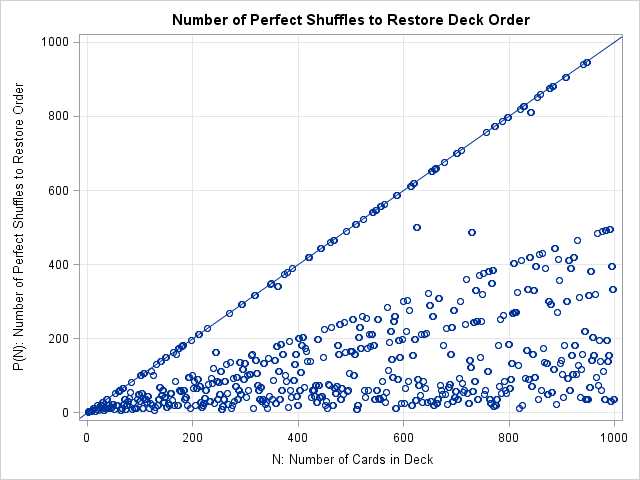### Coding the perfect riffle shuffle in SAS

The perfect riffle shuffle is easier to program than the Gilbert-Shannon-Reeds (GSR) model, which uses randomness to model how real people shuffle real cards. In a perfect riffle shuffle, you cut the deck exactly two equal parts. Then you interlace the cards from the top stack with the cards from the bottom stack. The new deck ordering is TBTBTB..., where T indicates a card from the top half of the original deck and B indicates a card from the bottom half.

There are actually two perfect riffle shuffles for an even number of cards: you can interlace the cards TBTBTB..., which is called an "out shuffle," or you can interlace the cards BTBTBT..., which is called an "in shuffle." For odd-numbered decks, there is also a choice of where to cut the deck: does the top part of the deck get the extra card or does the bottom part? My program always uses the "out shuffle" convention (the top card stays on top) and gives the top stack the extra card when there is an odd number of cards. Therefore, the shuffled deck looks like TBTB...TB for even-numbered decks and TBTB...TBT for odd-numbered decks. The following SAS/IML function takes a row vector that represents a card deck and performs a perfect riffle shuffle to reorder the cards in the deck:

```proc iml; start PerfectRiffle(deck); N = ncol(deck); /* send in deck as a row vector */ shuffle = j(1,N,.); /* allocate new deck */ nT = ceil(N/2); /* cut into two stacks; "Top" gets extra card when N odd */ nB = N - nT; /* nT = size of Top; nB = size of Bottom */ shuffle[, do(1,N,2)] = deck[,1:nT]; /* top cards go into odd positions */ shuffle[, do(2,N,2)] = deck[,nT+1:N]; /* bottom cards go into even positions */ return(shuffle); finish;   /* test the algorithm on a deck that has N = 10 cards */ origDeck = 1:10; deck = PerfectRiffle(origDeck); print (origdeck//deck)[r={"Orig Deck" "Perfect Riffle"}];```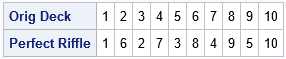The output shows one perfect riffle of a deck that has 10 cards that are originally in the order 1, 2, ..., 10.

### How many perfect riffle shuffles to restore order?

If you call the PerfectSuffle function repeatedly on the same deck, you can simulate perfect riffle shuffles. After each shuffle, you can test whether the order of the deck is in the original order. If so, you can stop shuffling. If not, you can shuffle again. The following SAS/IML loops test decks that contain up to 1,000 cards. The array nUntilRestore keeps track of how many perfect riffle shuffles were done for each deck size.

```decksize = 1:1000; /* N = 1, 2, 3, ..., 1000 */ nUntilRestore = j(1, ncol(decksize), .); /* the results vector */ do k = 2 to ncol(decksize); /* for the deck of size N */ origDeck = 1:decksize[k]; /* original order is 1:N */ deck = OrigDeck; /* set order of deck */ origOrder = 0; /* flag variable */ do nShuffles = 1 to decksize[k] until (origOrder); /* repeat shuffling */ deck = PerfectRiffle( deck ); /* shuffle deck */ origOrder = all(deck = origDeck); /* until the order of the cards are restored */ end; nUntilRestore[k] = nShuffles; /* store the number of shuffles */ end;   /* print the results for N=1,..,99 */ x = j(10, 10, .); x[2:100] = nUntilRestore[1:99]; /* present in 10x10 array */ rr = putn( do(0, 90, 10), "Z2."); /* each row has 10 elements */ cc = ("0":"9"); print x[r=rr c=cc L="Number of Perfect Shuffles to Restore Order"];   title "Number of Perfect Shuffles to Restore Deck Order"; call scatter(deckSize, nUntilRestore) grid={x y} other="lineparm x=0 y=0 slope=1;" label={"N: Number of Cards in Deck" "P(N): Number of Perfect Shuffles to Restore Order"} procopt="noautolegend";```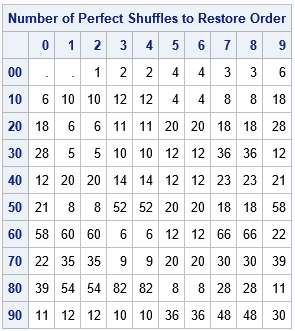The table shows the number of perfect riffle shuffles required for decks that have fewer than 99 cards. The results are in a 10x10 grid. The first row shows decks that have less than 10 cards, the second row shows sizes 10-19, and so on. For example, to find the result for a 52-card deck, move down to the row labeled "50" and over to the column labeled "2". The result in that cell is 8, which is the number of perfect shuffles required to restore a 52-card deck to its original order.

### Remarks on the number of perfect riffle shuffles

The graph at the top of this article shows the number of shuffles for decks that contain up to 1,000 cards. To me, the most surprising feature of the graph is the number of points that fall near diagonal lines of various rational slopes. The most apparent diagonal features are the lines whose slopes are approximately equal to 1, 1/2, and 1/3.

A complete mathematical description of this problem is beyond the scope of this blog article, but here are a few hints and links to whet your appetite.

• The integer sequence P(N) is related to the "multiplicative order of 2 mod 2n+1" in the On-Line Encyclopedia of Integer Sequences (OEIS). The encyclopedia includes a graph that is similar to the one here, but is computed for N ≤ 10,000. That integer sequence applies to the number of perfect riffle shuffles of an EVEN number of cards.
• The link to the OEIS shows a quick way to explicitly find P(N) for even values of N. P(N) is the smallest value of k for which 2k is congruent to 1 (mod N–1). For example, 8 is the smallest number for which 28 is congruent to 1 (mod 51) as you can compute explicitly: the value of mod(2**8, 51) is 1.
• The points in the graph for which P(N) = N-1 are all prime numbers: 2, 3, 5, 11, 13, 19, 29, 37, 53, 59, 61, 67, .... However, notice that not all prime numbers are on this list.

The post How many perfect riffle shuffles are required to restore a deck to its initial order? appeared first on The DO Loop.My colleague Robert Allison recently blogged about using the diameter of Texas as a unit of measurement. The largest distance across Texas is about 801 miles, so Robert wanted to find the set of all points such that the distance from the point to Texas is less than or equal to 801 miles.

Robert's implementation was complicated by the fact that he was interested in points on the round earth that are within 801 miles from Texas as measured along a geodesic. However, the idea of "thickening" or "inflating" a polygonal shape is related to a concept in computational geometry called the offset polygon or the inflated polygon. A general algorithm to inflate a polygon is complicated, but this article demonstrates the basic ideas that are involved. This article discusses offset regions for convex and nonconvex polygons in the plane. The article concludes by drawing a planar region for a Texas-shaped polygon that has been inflated by the diameter of the polygon. And, of course, I supply the SAS programs for all computations and images.

### Offset regions as a union of circles and rectangles

Assume that a simple polygon is defined by listing its vertices in counterclockwise order. (Recall that a simple polygon is a closed, nonintersecting, shape that has no holes.) You can define the offset region of radius r as the union of the following shapes:

• The polygon itself
• Circles of radius r centered at each vertex
• Rectangles of width r positions outside the polygon along each edge

The following graphic shows the offset region (r = 0.5) for a convex "house-shaped" polygon. The left side of the image shows the polygon with an overlay of circles centered at each vertex and outward-pointing rectangles along each edge. The right side of the graphic shows the union of the offset regions (blue) and the original polygon (red):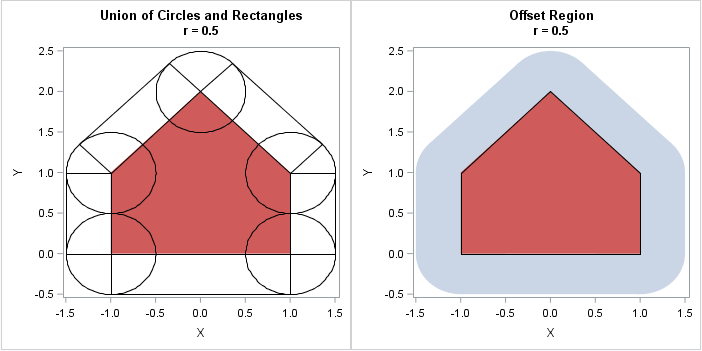The image on the right shows why the process is sometimes called an "inflating" a polygon. For a convex polygon, the edges are pushed out by a distance r and the vertices become rounded. For large values of r, the offset region becomes a nearly circular blob, although the boundary is always the union of line segments and arcs of circles.

You can draw a similar image for a nonconvex polygon. The inflated region near a convex (left turning) vertex looks the same as before. However, for the nonconvex (right turning) vertices, the circles do not contribute to the offset region. Computing the offset region for a nonconvex polygon is tricky because if the distance r is greater than the minimum distance between vertices, nonlocal effects can occur. For example, the following graphic shows a nonconvex polygon that has two "prongs." Let r0 be the distance between the prongs. When you inflate the polygon by an amount r > r0/2, the offset region can contain a hole, as shown. Furthermore, the boundary of the offset regions is not a simple polygon. For larger values of r, the hole can disappear. This demonstrates why it is difficult to construct the boundary of an offset region for nonconvex polygons.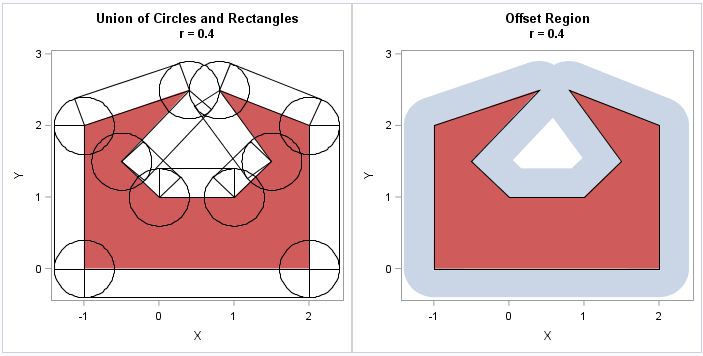### Inflating a Texas-shaped polygon

The shape of the Texas mainland is nonconvex. I used PROC GREDUCE on the MAPS.US data set in SAS to approximate the shape of Texas by a 36-sided polygon. The polygon is in a standardized coordinate system and has a diameter (maximum distance between vertices) of r = 0.2036. I then constructed the inflated region by using the same technique as shown above. The polygon and its inflated region are shown below.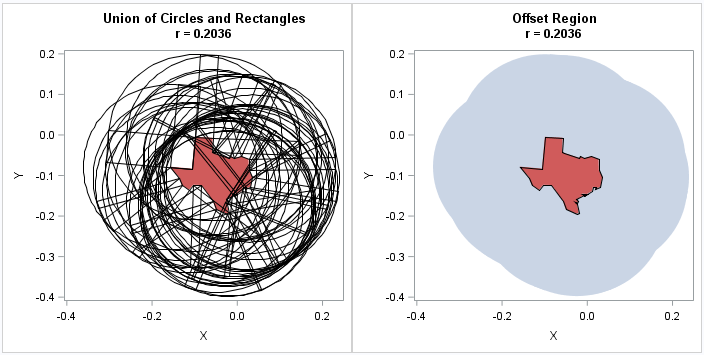The image on the left, which shows 36 circles and 36 rectangles, is almost indecipherable. However, the image on the right is almost an exact replica of the region that appears in Robert Allison's post. Remember, though, that the distances in Robert's article are geodesic distances on a sphere whereas these distances are Euclidean distances in the plane. For the planar problem, you can classify a point as within the offset region by testing whether it is inside the polygon itself, inside any of the 36 rectangles, or within a distance r of a vertex. That computation is relatively fast because it is linear in the number of vertices in the polygon.

I don't want to dwell on the computation, but I do want to mention that it requires fewer than 20 SAS/IML statements! The key part of the computation uses vector operations to construct the outward-facing normal vector of length r to each edge of the polygon. If v is the vector that connects the i_th and (i+1)_th vertex of the polygon, then the outward-facing normal vector is given by the concise vector expression r * (v / ||v||) * M, where M is a rotation matrix that rotates by 90 degrees. You can download the SAS program that computes all the images in this article.

In conclusion, you can use a SAS program to construct the offset region for an arbitrary simple polygon. The offset region is the union of circles, rectangles, and the original polygon, which means that it is easy to test whether an arbitrary point in the plane is in the offset region. That is, you can test whether any point is within a distance r to an arbitrary polygon.

The post Offset regions: Find all points within a specified distance from a polygon appeared first on The DO Loop.